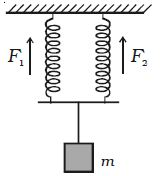# Explain When two Springs are Connected in Parallel

When two Springs are Connected in Parallel:

Two springs of spring factors k1 and k2 are suspended from a rigid support as shown in Figure. A load m is attached to the combination.

Let the load be pulled downwards through a distance y from its equilibrium position. The increase in length is y for both the springs but their restoring forces are different.If F1 and F2 are the restoring forces

F1 = – k1y,        F2 = – k2y

Total restoring force = (F1 + F2) = – (k1 + k2) y

So, time period of the body is given by;

T = 2π √[m/( k1 + k2)]

If, k1 = k2 = k; then,

T = 2π √(m/2k)

And frequency n = 1/2π √(2k/m)